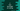# C program to find the length of a string### C program to find the length of a string :

In this tutorial, we will learn how to find the length of a string using C programming language. We will check two different ways to find the length of a string. The string will be provided by the user. Let’s take a look at the program :

### C program using for loop :

``````#include <stdio.h>

int main()
{
//1
char inputString;
int length = 0;
int i;

//2
fgets(inputString, 100, stdin);

//3
for (i = 0; inputString[i] != '\n'; i++)
{
length += 1;
}

//4
printf("Length of the string is %d \n", length);
}``````

### Explanation :

1. Create one char array to store the string. Create one integer length to store the size of the string and one integer i to use in the for loop.
2. Ask the user to enter a string and store it in the variable inputString.
3. Run one for loop and read each character one by one. This loop will run till the current character is \n or new line i.e. the last character of the string. Increment the variable length by 1 for each character.
4. Print the length of the string. The variable length holds the size of the string.

### C program to find the length using the built-in function :

We can also find the length of the string by using the strlen() function.To use it, you need to include string.h on top. It also counts the new line character \n, so we are decrementing 1 from the result.Let’s take a look :

``````#include <stdio.h>
#include <string.h>

int main()
{
char inputString;

fgets(inputString, 100, stdin);

printf("Length of the string is %d \n", strlen(inputString) - 1);
}``````

### Sample Output :

``````Enter your string : Hello World
Length of the string is 11

Enter your string : this is a string
Length of the string is 16